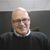# New to Qlik Sense

If you’re new to Qlik Sense, start with this Discussion Board and get up-to-speed quickly.

Announcements
Skip the ticket, Chat with Qlik Support instead for instant assistance.
cancel
Showing results for
Did you mean:Partner - Creator III

## Result of any Date+X days in qlik sense

hi,

considering the date as filter and X as another filter.

If i click on date(07/22/2017) and X as 4, i need a result of july22 to july 26.

tried with expression

Sum({<[dimension_field]={'=[date]<= [date]+x'}>}metric)

but not getting proper result.

can anyone say the correct expression for this.

thanks1 Solution

Accepted SolutionsPartner - Champion III

At the least you need to fix the quotation marks to be double quotation as you are trying to do a search which requires double quotation in the later versions of Qlik Sense. In addtion I believe that you want all dates between [date] and up to [date]+x and then you have to fix that too:

Sum( {<[dimensions_field]={">=\$(=[date])<=\$(=Date([date]+X))"}>} metric )

Or something more like this:

Sum({<[date]={">=\$(=[date])<=\$(=Date([date]+X))"},X=>} [Sales Qty] )

2 RepliesPartner - Champion III

At the least you need to fix the quotation marks to be double quotation as you are trying to do a search which requires double quotation in the later versions of Qlik Sense. In addtion I believe that you want all dates between [date] and up to [date]+x and then you have to fix that too:

Sum( {<[dimensions_field]={">=\$(=[date])<=\$(=Date([date]+X))"}>} metric )

Or something more like this:

Sum({<[date]={">=\$(=[date])<=\$(=Date([date]+X))"},X=>} [Sales Qty] )Partner - Contributor II

Hi !!

Try this!

sum({<datefield={" >=\$(=max(datefield))<=\$(=num(max(datefield))+X) "}>}sales)

Hope this works fine!!Tags
Community Browser